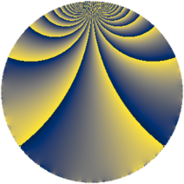# Properties

 Label 195.2.vLevel $195$ Weight $2$ Character orbit 195.v Rep. character $\chi_{195}(4,\cdot)$ Character field $\Q(\zeta_{6})$ Dimension $32$ Newform subspaces $1$ Sturm bound $56$ Trace bound $0$

# Related objects

## Defining parameters

 Level: $$N$$ $$=$$ $$195 = 3 \cdot 5 \cdot 13$$ Weight: $$k$$ $$=$$ $$2$$ Character orbit: $$[\chi]$$ $$=$$ 195.v (of order $$6$$ and degree $$2$$) Character conductor: $$\operatorname{cond}(\chi)$$ $$=$$ $$65$$ Character field: $$\Q(\zeta_{6})$$ Newform subspaces: $$1$$ Sturm bound: $$56$$ Trace bound: $$0$$

## Dimensions

The following table gives the dimensions of various subspaces of $$M_{2}(195, [\chi])$$.

Total New Old
Modular forms 64 32 32
Cusp forms 48 32 16
Eisenstein series 16 0 16

## Trace form

 $$32 q - 20 q^{4} + 16 q^{9} + O(q^{10})$$ $$32 q - 20 q^{4} + 16 q^{9} + 2 q^{10} - 12 q^{11} + 8 q^{14} - 6 q^{15} - 28 q^{16} - 30 q^{20} - 4 q^{25} + 52 q^{26} - 24 q^{29} + 4 q^{30} - 2 q^{35} + 20 q^{36} + 4 q^{40} - 36 q^{41} + 12 q^{45} - 48 q^{46} - 28 q^{49} + 54 q^{50} - 40 q^{51} + 24 q^{55} - 56 q^{56} + 84 q^{59} - 32 q^{61} + 136 q^{64} + 20 q^{65} + 8 q^{66} - 24 q^{69} + 12 q^{71} + 40 q^{74} - 16 q^{75} + 48 q^{76} - 104 q^{79} + 66 q^{80} - 16 q^{81} - 48 q^{84} - 54 q^{85} - 48 q^{89} + 4 q^{90} + 12 q^{91} - 8 q^{94} + 12 q^{95} + O(q^{100})$$

## Decomposition of $$S_{2}^{\mathrm{new}}(195, [\chi])$$ into newform subspaces

Label Dim $A$ Field CM Traces $q$-expansion
$a_{2}$ $a_{3}$ $a_{5}$ $a_{7}$
195.2.v.a $32$ $1.557$ None $$0$$ $$0$$ $$0$$ $$0$$

## Decomposition of $$S_{2}^{\mathrm{old}}(195, [\chi])$$ into lower level spaces

$$S_{2}^{\mathrm{old}}(195, [\chi]) \cong$$ $$S_{2}^{\mathrm{new}}(65, [\chi])$$$$^{\oplus 2}$$